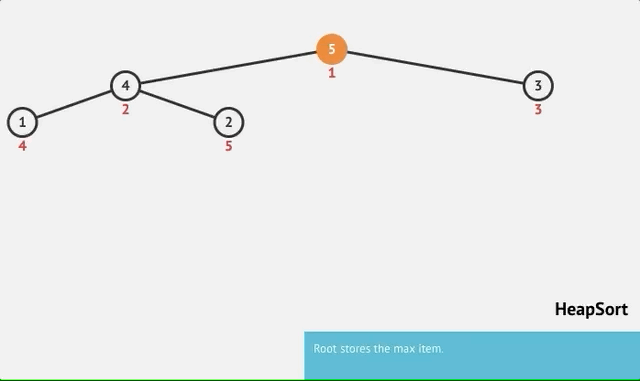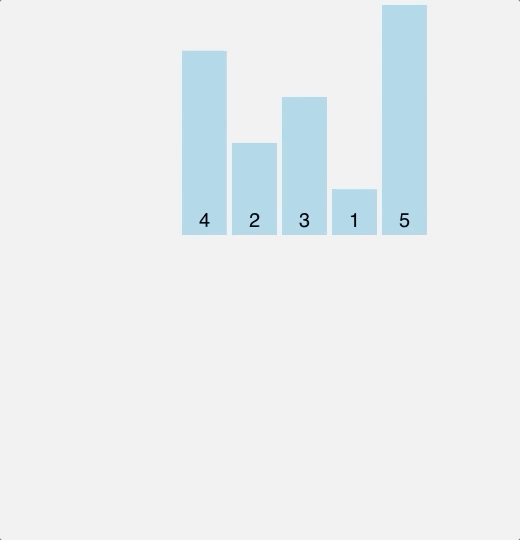# Sorting Algorithms with Javascript (Part 2)

##### Nov 04, 2018

I’m going to show Javascript implementations of three more sorting algorithms:

• Quick sort
• Heap sort
• Counting sort

Again, this is not intended to be an in-depth explanation on the ins and outs of how the algorithms work and their performance. If you’d rather read about that, here’s a nice resource I found: Sorting Algorithms

To keep things simple, I’ll be sorting a simple list `list` having only 5 elements `[4, 2, 3, 1, 5]`.

## Quick Sort

Like merge sort, this algorithm takes a divide-and-conquer approach. It works by picking a pivot and dividing the list in relation to that pivot: all elements greater than the pivot go to the right of the pivot, and all elements less than or equal to the pivot go to the left of the pivot. Elements on both sides are quick sorted, and this is repeated until the complete list is sorted.

#### Visual

The visual on this wasn’t very clear in illustrating how the algorithm actually works so here’s a video instead.

#### Code

``````function quickSort(list) {
if (list.length <= 1) {
return list
} else {
const left = []
const right = []
const sorted = []
const pivot = list.pop() // we're picking the last item to act as the pivot
const length = list.length

for (let i = 0; i < length; i++) {
if (list[i] <= pivot) {
left.push(list[i])
} else {
right.push(list[i])
}
}

return sorted.concat(quickSort(left), pivot, quickSort(right))
}
}

const list = [4, 2, 3, 1, 5]

const sorted = quickSort(list)

console.log(sorted)
``````

## Heap Sort

This algorithm takes advantage of a data structure known as a binary-heap. Heap sort works by creating a Max heap to sort the elements in ascending order, then swapping the root node with the last node, and deleting the sorted node from the heap every time this is done.

#### Visual#### Code

``````// create max heap
function maxHeap(input, i) {
const left = 2 * i + 1
const right = 2 * i + 2
let max = i

if (left < arrLength && input[left] > input[max]) {
max = left
}

if (right < arrLength && input[right] > input[max]) {
max = right
}

if (max != i) {
swap(input, i, max)
maxHeap(input, max)
}
}

function swap(input, indexA, indexB) {
const temp = input[indexA]

input[indexA] = input[indexB]
input[indexB] = temp
}

function heapSort(input) {
arrLength = input.length

for (let i = Math.floor(arrLength / 2); i >= 0; i -= 1) {
maxHeap(input, i)
}

for (i = input.length - 1; i > 0; i--) {
swap(input, 0, i)
arrLength--

maxHeap(input, 0)
}
return
}

let arrLength

const list = [4, 2, 3, 1, 5]

const sorted = heapSort(list)

console.log(list)
``````

## Counting Sort

You’ll find counting sort to be rather unique compared to the algorithms we’ve covered so far. This is because it does not compare elements while sorting. It works based on numeric keys. It does this by creating a counting array, then using it to determine an element’s correct position.

#### Visual#### Code

``````function countingSort(list, min, max)
{
let i
let z = 0
const count = []

for (i = min; i <= max; i++) {
count[i] = 0
}

for (i=0; i < list.length; i++) {
count[list[i]]++
}

for (i = min; i <= max; i++) {
while (count[i]-- > 0) {
list[z++] = i
}
}
return list
}

const list = [4, 2, 3, 1, 5]
const min = Math.min(...list)
const max = Math.max(...list)
const sorted = countingSort(list, min, max)

console.log(sorted)
``````